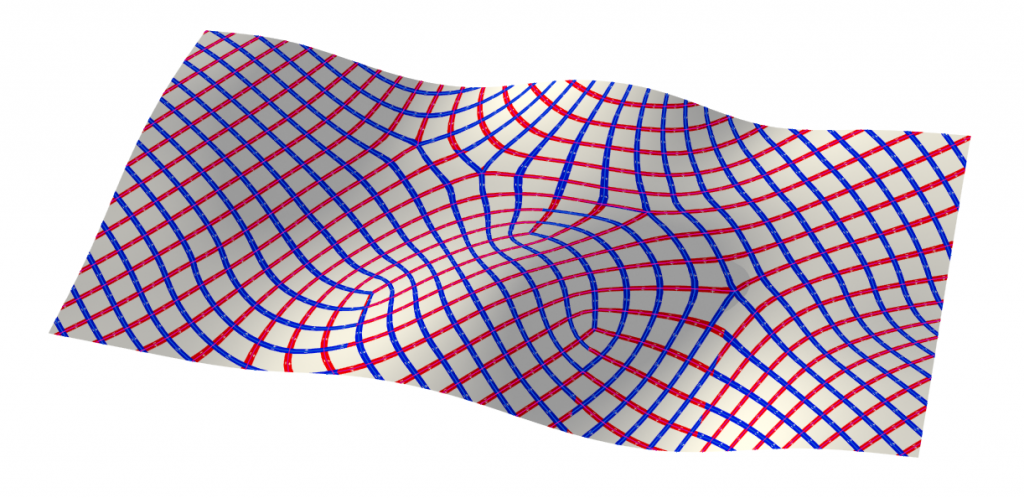Previous | Next --- Slide 23 of 46
Back to Lecture Thumbnailsljelenak

I feel like we talk a lot about triangle meshes, and then a lot about polygon meshes, in which cases are each used? Is one more common in one application, and is the other better for other cases (maybe algorithms and such)?keenan

@ljelenak In practice, across all of graphics, you pretty much see either (i) pure triangle meshes or (ii) quad-dominant meshes, i.e., meshes with mostly quads and just a few triangles/pentagons/whatever.

Triangle meshes are used for many applications because you just want to treat one common case---the chief example from our class being rasterization, where the driver dices up polygons (and points, and lines, and ...) into triangles before drawing them. This way, the hardware only has to implement algorithms for triangles and nothing else. Likewise, many algorithms across rendering, geometry, and animation use triangles and nothing else, because triangles are sufficiently rich to represent any kind of geometry, and then the algorithm only has to consider one simple case. In this sense, triangles are kind of to surfaces what pixels are to images.

Quad, or more realistically, quad-dominant meshes also show up in a fair number of algorithms for some natural geometric and computational reasons. For one thing, every point of a smooth surface has two principal directions, i.e., two directions along which the surface bends the most and the least, and tracing out these principal directions along the surface sort of naturally splits it up into a network of quads (called the principal curvature network):In fact, the directions in this network just so happen to always be orthogonal. From the point of view of geometric approximation, it is therefore natural to describe surfaces as collections of quads with (nearly) 90-degree angles; in fact, one can show that curvature line-aligned quads give the best asymptotic approximation for smooth surfaces (i.e., as the number of elements goes to infinity). Another good motivation for using quads is that they naturally lend themselves to really nice subdivision surfaces (Catmull-Clark), especially in regular regions where the limit surface is just a bicubic patch and is hence super easy to evaluate. These two reasons combined lead folks like Pixar to use quad-dominant meshes for many of their models: the edges nicely follow features on the surface, and the limit surface under subdivision is well-behaved. There are some people who also prefer quads for finite element problems, because you have natural basis functions associated with quads: bilinear functions. However, if you really dig into this question you will find a lot of controversy about whether bilinear elements are really better than linear elements in terms of the overall price/performance ratio of the finite element solver (i.e., how much accuracy you get for a fixed amount of computation).